# Lesson 4 Linear Equations Solving OneStep Equations WarmUp

• Slides: 15Lesson 4 Linear Equations Solving One-Step EquationsWarm-Up Evaluate each expression for x = − 4. 1. x+7 2. 3 x − 1 3. −x 4. x 2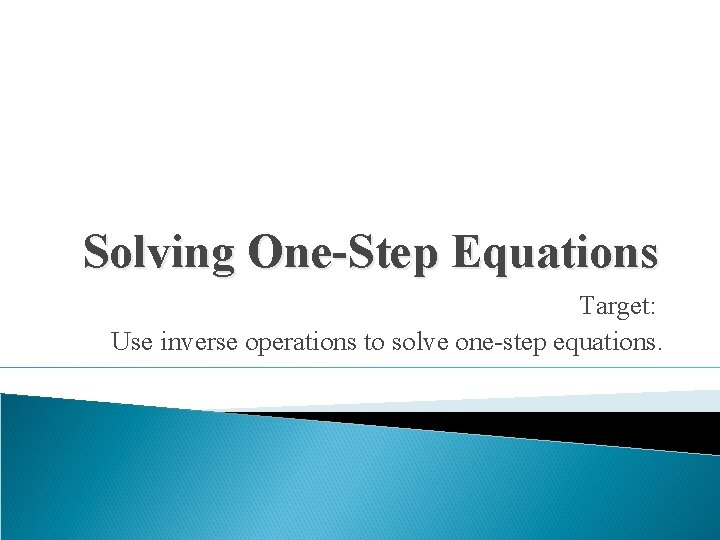Solving One-Step Equations Target: Use inverse operations to solve one-step equations.The Properties of Equality For any numbers a, b and c: � If a = b then a + c = b + c (Addition Property of Equality) � If a = b then a – c = b – c (Subtraction Property of Equality) � If a = b then a ∙ c = b ∙ c (Multiplication Property of Equality) � If a = b then a ÷ c = b ÷ c (Division Property of Equality)Vocabulary � Zero Pair: One positive integer chip paired with one negative integer chip. � Inverse Operation: Operations that undo each other.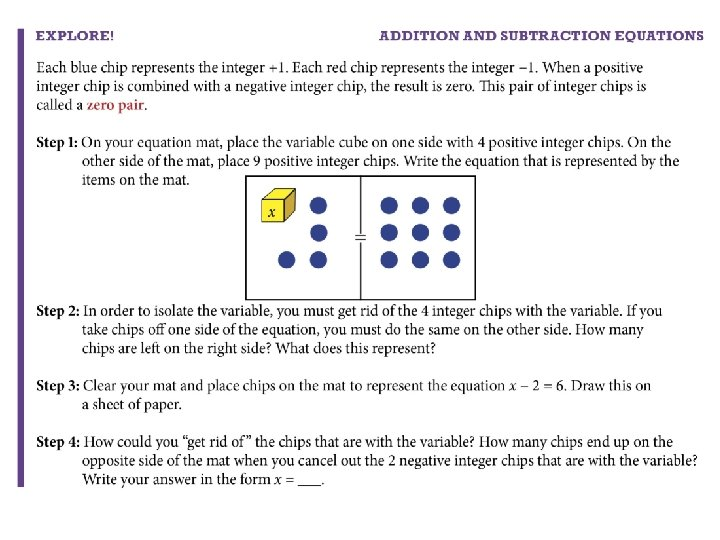Example 1 ab Solve for x. Check your solution. �x – 6 = 22 +6 +6 x = 28 28 – 6 = 22 � 8 x = 88 8 8 x = 11 8(11) = 88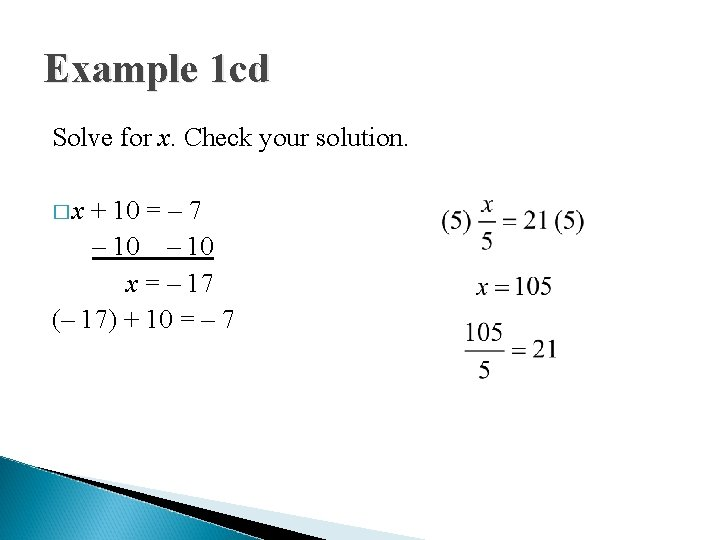Example 1 cd Solve for x. Check your solution. �x + 10 = – 7 – 10 x = – 17 (– 17) + 10 = – 7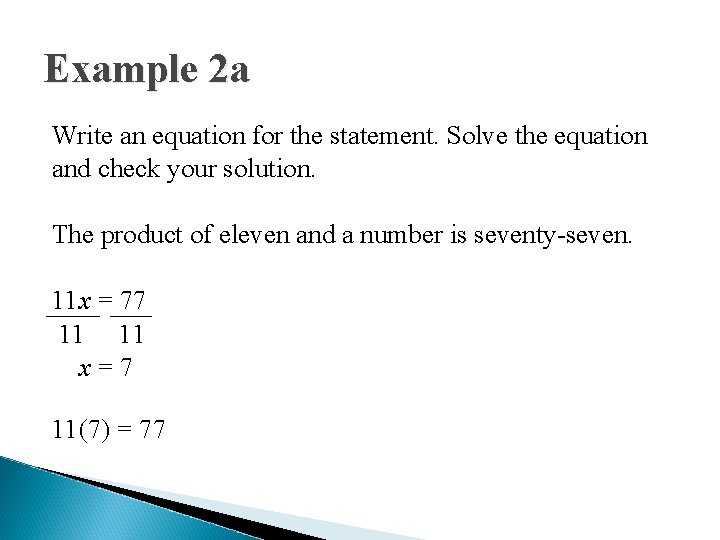Example 2 a Write an equation for the statement. Solve the equation and check your solution. The product of eleven and a number is seventy-seven. 11 x = 77 11 11 x=7 11(7) = 77Example 2 b Write an equation for the statement. Solve the equation and check your solution. The sum of a number and 52 is 98. x + 52 = 98 – 52 x = 46 46 + 52 = 98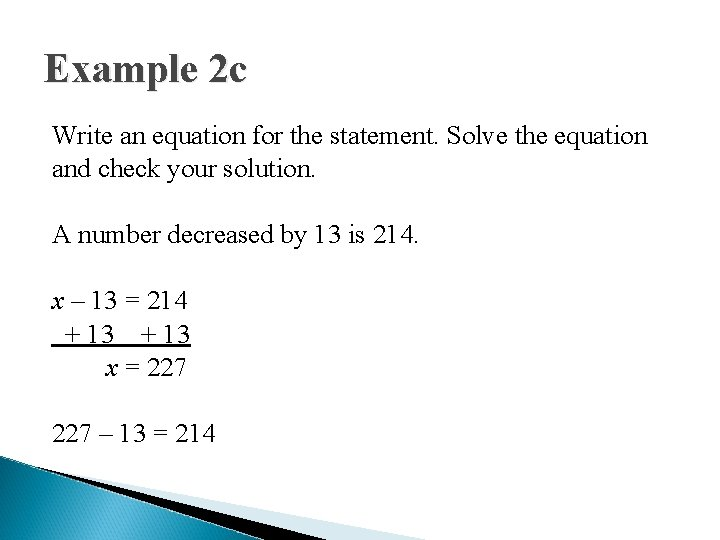Example 2 c Write an equation for the statement. Solve the equation and check your solution. A number decreased by 13 is 214. x – 13 = 214 + 13 x = 227 – 13 = 214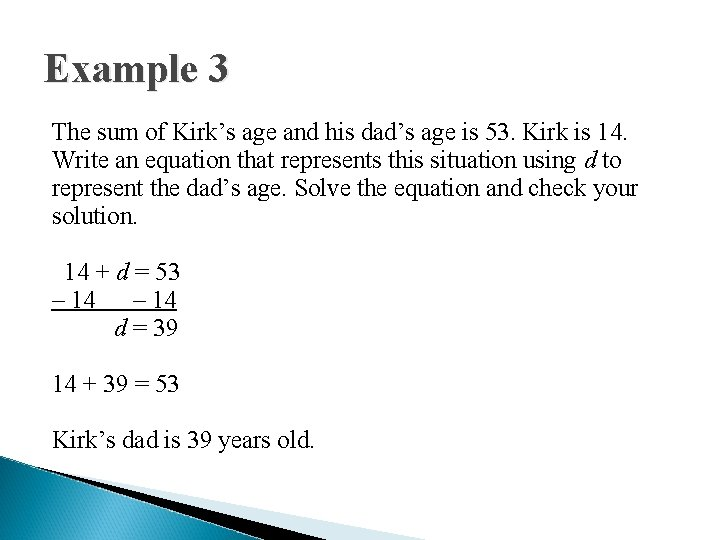Example 3 The sum of Kirk’s age and his dad’s age is 53. Kirk is 14. Write an equation that represents this situation using d to represent the dad’s age. Solve the equation and check your solution. 14 + d = 53 – 14 d = 39 14 + 39 = 53 Kirk’s dad is 39 years old.Exit Problems Solve each equation for x. 1. x – 70 = 138 2. 3. 4. 11 x = 66Communication Prompt How are manipulatives helpful when solving one-step equations?